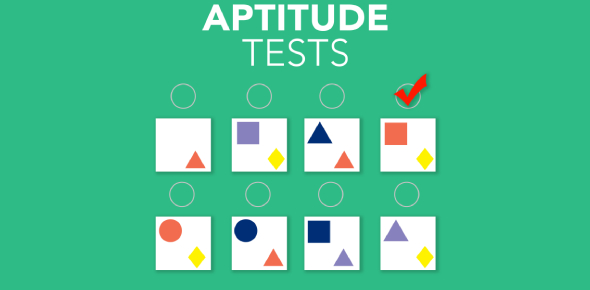# Can You Pass This Hardest Aptitude Test?

10 Questions | Total Attempts: 3235SettingsAn Aptitude Test is a methodical way of testing a candidate's mental abilities which include analytical and reasoning skills needed in a job or attending any competitive exams. Basically, this test is used in the pre-employment selection process as an assessment tool. Take this quiz on Aptitude and prove your worth. Read the questions carefully and answer. So, let's try out the quiz. All the best!

Related Topics
• 1.
If the radius of a circle is diminished by 10%, then its area is diminished by:
• A.

10%

• B.

19%

• C.

20%

• D.

36%

• 2.
At what time after 4.00 p.m. is the minute's hand clock exactly aligned with the hour hand?
• A.

4:21:49.5

• B.

4:27:49.5

• C.

3:21:49.5

• D.

4:21:44.5

• 3.
A shopkeeper sold a T.V set for Rs. 17,940 with a discount of 8% and earned a profit of 19.6%. What would have been the percentage of profit earned if no discount was offered?
• A.

24.8%

• B.

25%

• C.

26.4%

• D.

Cannot be determined

• E.

None of these

• 4.
If (2x-y)=4 then (6x-3y) =?
• A.

15

• B.

12

• C.

18

• D.

10

• 5.
What is the missing number in this series? 8 2 14 6 11 ? 14 6 18 12
• A.

16

• B.

9

• C.

15

• D.

6

• 6.
A person has 4 coins each of different denomination. What the number of different sums of money  the person can form(using one or more coins at a time)?
• A.

16

• B.

15

• C.

12

• D.

11

• 7.
A cow is tethered in the middle of the field with a 14 feet long rope. If the cow grazes 100 sq. ft. per dy, then approximately what time will be taken by the cow to graze the whole field?
• A.

2 days

• B.

6 days

• C.

18 days

• D.

24 days

• E.

None of these

• 8.
The two colours seen at the extreme ends of the pH chart are:
• A.

Red and blue

• B.

Red and green

• C.

Green and blue

• D.

Orange and green

• 9.
If the value of x lies between 0 and 1 which of the following2 is the largest?
• A.

X

• B.

X2

• C.

-x

• D.

1/x

• 10.
Thirty men take 20 days to complete a job working 9 hours a day. How many hours a day should 40 men  work to complete the job?
• A.

8 hrs

• B.

71/2 hrs

• C.

7 hrs

• D.

9 hrs